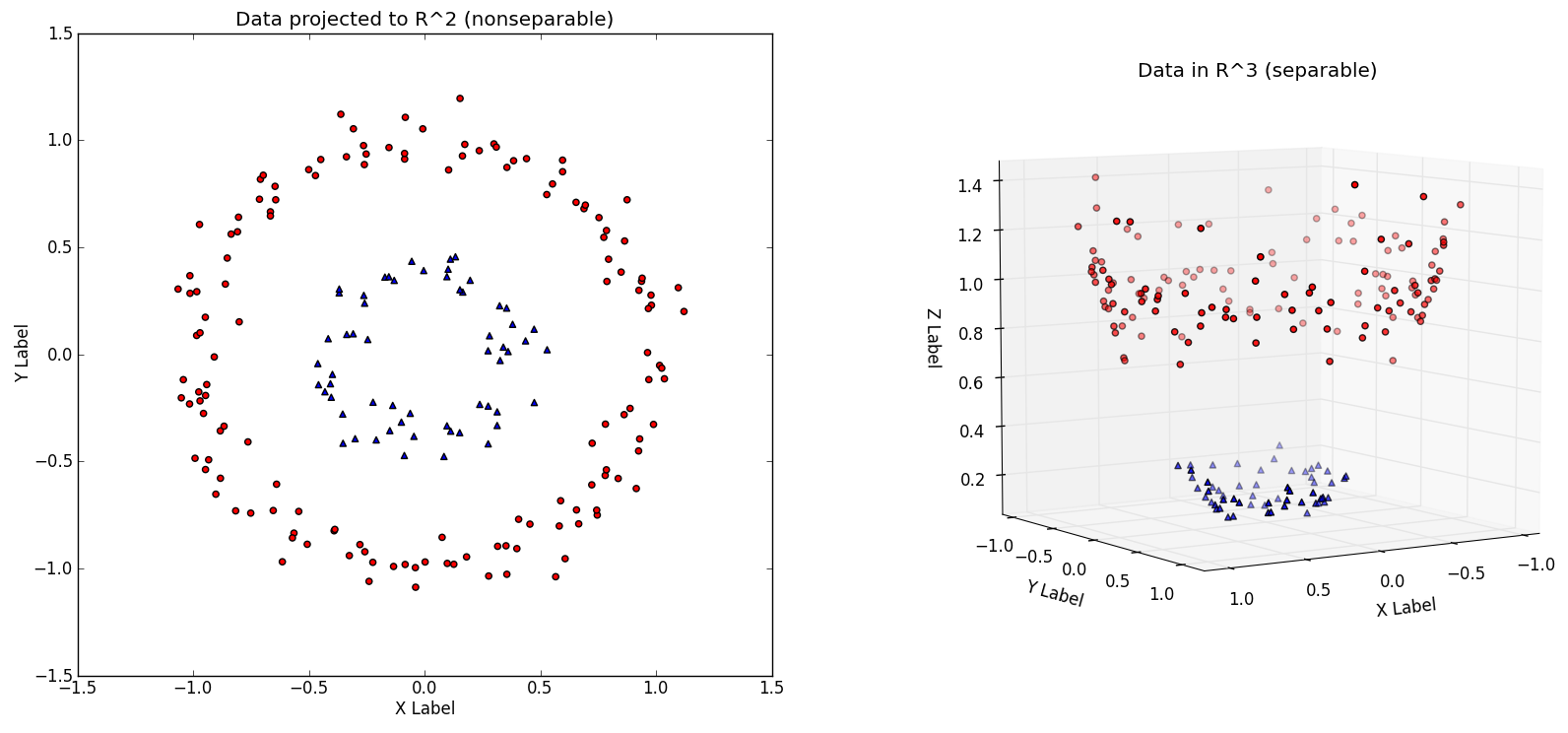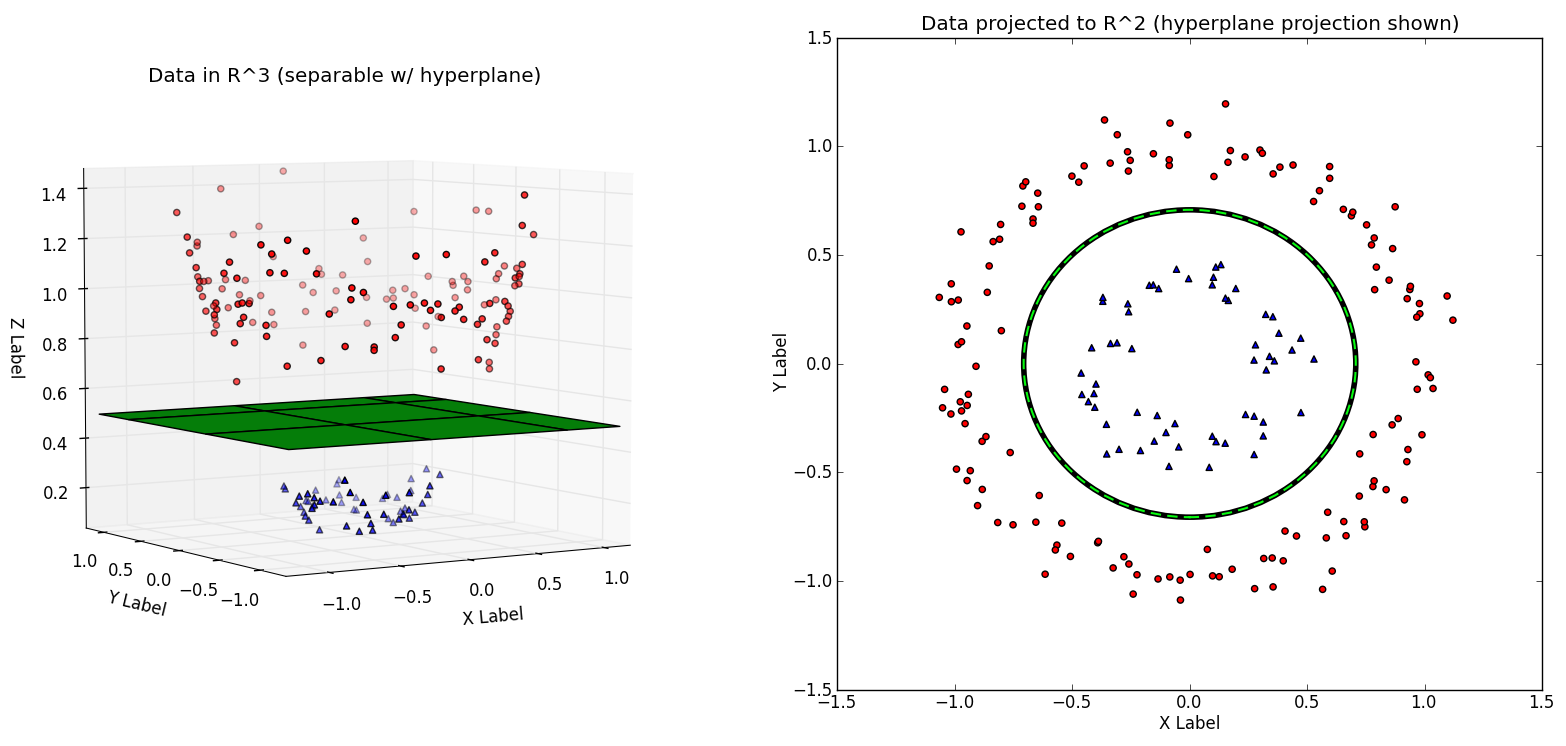# Machine learning for dummies – Support Vector Machines

Support Vector Machines, it sounds scary. Might be a name of some horror movie. Revenge of Support Vector Machines. But do not worry, for us dummies it’s not so complicated. I promise I will not use any intimidating words such as Lagrangian or Mercer’s Theorem. To make it even simpler, I will just talk about classification between two classes, as if we were trying to decide if a letter is an ‘A’ or not (please read previous post if you do not know what I am talking about).

SVMs are really similar to Logistic Regression, they try to find linear (hyper)plane that would separate our classes, one class on one side of the plane, the other on the other side. There are two important differences from Logistic Regression. The first one is that SVMs try to find plane that is as far as possible from both classes. But that’s not so interesting. When trying to classify letters, our problem is that most likely you can not find any such plane in our 784 dimensional space (28×28 pixels) that would separate A’s from other letters.

Imagine that we got to situation on the image below. For simplicity only in 2 dimension, as if our letters had only two pixels. (images taken from Everything You Wanted to Know about the Kernel Trick)Imagine that our A’s are in the middle surrounded by their enemies, other letters. I just can not draw a straight line to separate them.

But I can escape to another dimension by creating a third feature like \$latex x_1^2 + x_2^2\$ (image on the right). Now I can separate the two classes. Look ma, I can draw a circle using a linear function!While I can manually find a new feature that would separate the classes, I am to lazy to do that. It’s called Machine Learning, I want the machine to do it for me. SVMs can hep me with that. I can ask them to use a “kernel” which will make my model non-linear. Here is how it works, hopefully sufficiently dummified.

Imagine I have 20,000 training samples. The first thing the model does is that it remembers them all. It just shamelessly stores all 20k samples. Now imagine I have a new sample coming to the model. The model calculates distances from my 20k training samples to my new sample which results in 20k new features. First feature is the distance to the first sample in my training set, second is the distance to the second sample etc. Suddenly I have twenty-thousand-dimensional space and it’s much more likely that I will find a linear plane that would separate both classes. This method intuitively makes sense. Points representing A’s are likely to be near other point’s representing A’s so if we somehow incorporate distance into the model, it should get better result. In more clever sounding words, if I project the plane form 20k dimensional space back to my original 784 dimensional space, I can get highly nonlinear boundary.

Now a bit less dummified version (dummified is a word, I swear). Not all 20k training samples are used, only the most interesting ones (they are called Support Vectors hence the name). So the space we are using has less than 20k dimensions. Moreover the distance is not necessary a distance, it’s just some function that takes two points (vectors) as an input and returns a number (new feature). This function is called kernel and we pick it when configuring the classifier – Gaussian (rbf) is the most common. And while the method sounds complicated and slow, it uses some mathematical shortcuts so it’s relatively fast. Unfortunately it does not scale well with number of training samples.

Let’s see how the method looks in the code.

``````clf = svm.SVC(C=10, gamma=0.01, cache_size=2000)
``````

The rest of the code is the same as the last time, we’ve just changed the classifier. We see our favorite (hyper)parameter C. There is also a new parameter ‘gamma’ which configures the kernel. Smaller gamma makes our Support Vectors reach further and generalize more.

So what are the results? We got to 99.7% accuracy on the training set. It’s not surprising, the model actually remembers some of the training samples. If we call ‘clf.support_.shape’ it will tell us that it remembers 8,670 samples out of 20,000. It means that our model has to learn 8,670 parameters for each classified class. Let’s count how many numbers the model needs to remember. It stores 8,670 samples each of them having 28×28=784 values. We are trying to classify 10 letters so the model learns 10×8,670 parameters. (Actually 9×8,670, but let’s not go there.) All in all, the model consists of almost 7,000,000 parameters (numbers) most of it in form of Support Vectors picked from training samples. The training takes less than 3 minutes on my laptop.

What are the results on the validation set, on the samples that the model has not seen? It is 87%. The nonlinearity of the model gave us five more percent when compared to Logistic Regression. It’s great. The author of notMNIST set says that the set has ~6,5% label error rate so we need six more percent to get perfect. To confirm that I could not resist and run the classification on test set, which is much cleaner. I got 93% accuracy. Like 93% on this crazy images? Incredible. We still have further 6-7% to go and I am afraid we will have to resort to neural networks to get better results.

## 2 thoughts on “Machine learning for dummies – Support Vector Machines”

1.Martin Chalupa

Very nice article. I’m little bit puzzled with calculation of stored parameters within model. I counted 8670 samples * 784 values * 10 letter ~ 70 000 000 but you write 7 000 000. Can you explain that, please?

2.Lukáš Křečan

Good question. In scikit implementation there is one model for all classes (letters). In other words, the support vectors are shared and only the linear equation parameters are different for each letter. So it’s 8,670*784 + 10*8,670. The classification works like this. New sample comes, distance from all support vectors is calculated. It results in 8.7k new features. We then multiply this feature vector with parameters for each letter which gives us a number. We then pick the letter with the largest number.